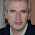1 mar. 2012

NIT: Fatty acids study in R - Part 001

This time I´m going to use my own data to develop a model to predict some fatty acid in the solid fat (pork).
Samples had been analyzed in a NIT (Near Infrared Transmittance) instrument. The range of the wavelengths is from 850 to 1048 nm (100 data points). This area cover usually second overtones.
I am quite novice in R, and it takes some time (in my case) to have the data organize in R to starts working with it.
We have together the X matrix (NIT absorbance’s) with the Y matrix (constituents). First of all was to import the”txt” file and create a data frame.
The TXT file has been exported from the software of the instrument.

First thing to do is organize the data frame and plot the raw spectra of the samples:
NIT<-as.matrix(fat[,7:106])
C16_0<-fat[,1]
C16_1<-fat[,2]
C18_0<-fat[,3]
C18_1<-fat[,4]
C18_2<-fat[,5]
C18_3<-fat[,6]
fattyac<-data.frame(C16_0=I(C16_0),C16_1=I(C16_1),C18_0=I(C18_0),
C18_1=I(C18_1),C18_2=I(C18_2),C18_3=I(C18_3),NIT=I(NIT))
names(fattyac)
#  "C16_0" "C16_1" "C18_0" "C18_1" "C18_2" "C18_3" "NIT"
class(fattyac\$NIT)
wavelengths=seq(from=850,to=1048,by=2)
matplot(wavelengths,t(fattyac\$NIT),lty=1,type="l",
pch=NULL,xlab="nm",ylab="abs")

We can see a high scatter, so a remove scatter pretreatment is necessary.
Let´s try the MSC.

For a better understanding of the MSC antiscatter pretreatment, see the post:
Multiple Scatter Correction (MSC)

library(ChemometricsWithR)
fat_msc<-msc(NIT)
matplot(wavelengths,t(fat_msc),lty=1,type="l",
+ pch=NULL,xlab="nm",ylab="abs")

Now we can create the data frame again ewith the spectra treated eith the MSC indeed the raw spectra:
fattyac_msc<-data.frame(C16_0=I(C16_0),C16_1=I(C16_1),
C18_0=I(C18_0),C18_1=I(C18_1),C18_2=I(C18_2), C18_3=I(C18_3),NITmsc=I(fat_msc))

We will use this data frame in the next posts.

If you want to follow this tutorial, please send me an e_mail. I´ll send you the “txt” file attached.

2 comentarios:

1.Are the raw data available online so that we can run the analyses ourselves?

Niall Bolger

1.Dear Niall,
Thanks for the comment.
Send me an e-mail (cuesta_joseramon@yahoo.es), I´ll reply with the "txt" file attached.